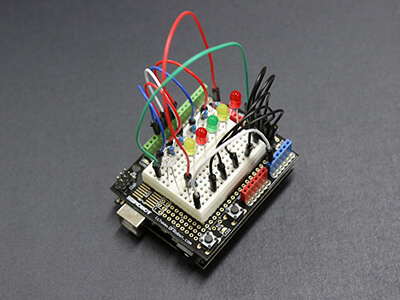Learning Objective

In this lesson we will learn about basic electric circuits and how they can be represented by circuit diagrams.

Learning Outcomes

By the end of this lesson you will be able to:

• Describe an electric circuit and list four common features of all electric circuits.

• Describe how electric circuits can be represented by circuit diagrams.

• Compare the arrangement of components in series circuits and parallel circuits.

## Contents

1 | Electric Circuits

2 | Circuit Diagrams

3 | Series Circuits and Parallel Circuits

4 | Summary

BUY THE YEAR 9 PHYSICS WORKBOOK(Image: manseok, Pixabay)